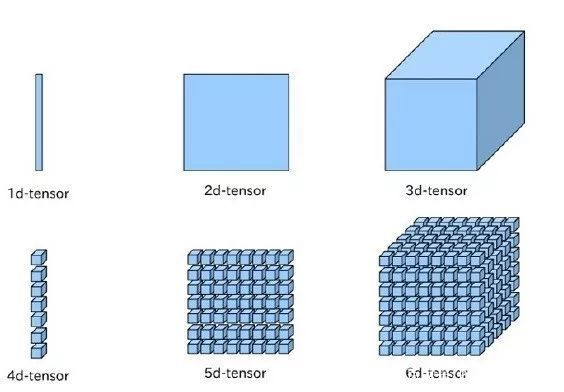# 如何用Paddle Fluid API搭建一个简单的神经网络？

2019/05/28 08:42

• Paddle Fluid 有哪些核心概念

• 如何在 Paddle Fluid 中定义运算过程

• 如何使用 executor 运行 Paddle Fluid 操作

• 如何从逻辑层对实际问题建模

• 如何调用 API（层，数据集，损失函数，优化方法等等）

Paddle Fluid 和其它主流框架一样，使用 Tensor 数据结构来承载数据。Tensor 可以简单理解成一个多维数组，一般而言可以有任意多的维度。不同的 Tensor 可以具有自己的数据类型和形状，同一 Tensor 中每个元素的数据类型是一样的，Tensor 的形状就是 Tensor 的维度。Paddle Fluid 中存在三种特殊的 Tensor：

1. 模型中的可学习参数

Paddle Fluid 中可以通过 fluid.layers.create_parameter 来创建可学习参数：

w = fluid.layers.create_parameter(name="w",shape=,dtype='float32')

import paddle.fluid as fluid
y = fluid.layers.fc(input=x, size=128, bias_attr=True)

2. 输入输出 Tensor

Paddle Fluid 中使用 fluid.layers.data 来接收输入数据，fluid.layers.data 需要提供输入 Tensor 的形状信息，当遇到无法确定的维度时，相应维度指定为 None 或 -1，如下面的代码片段所示：

import paddle.fluid as fluid
#定义x的维度为[3,None]，其中我们只能确定x的第一的维度为3，第二个维度未知，要在程序执行过程中才能确定
x = fluid.layers.data(name="x", shape=[3,None], dtype="int64")
#batch size无需显示指定，框架会自动补充第0维为batch size，并在运行时填充正确数值
a = fluid.layers.data(name="a",shape=[3,4],dtype='int64')
#若图片的宽度和高度在运行时可变，将宽度和高度定义为None。
#shape的三个维度含义分别是：channel、图片的宽度、图片的高度
b = fluid.layers.data(name="image",shape=[3,None,None],dtype="float32")

3. 常量 Tensor

Paddle Fluid 通过 fluid.layers.fill_constant 来实现常量 Tensor，用户可以指定 Tensor 的形状，数据类型和常量值。代码实现如下所示：

import paddle.fluid as fluid
data = fluid.layers.fill_constant(shape=, value=0, dtype='int64')

print data

name: "fill_constant_0.tmp_0"
type {
type: LOD_TENSOR
lod_tensor {
tensor {
data_type: INT64
dims: 1
}
}
}
persistable: false

Paddle Fluid 中，所有对数据的操作都由 Operator 表示，您可以使用内置指令来描述它们的神经网络。为了便于用户使用，在 Python 端，Paddle Fluid 中的 Operator 被一步封装入 paddle.fluid.layers，paddle.fluid.nets 等模块。这是因为一些常见的对 Tensor 的操作可能是由更多基础操作构成，为了提高使用的便利性，框架内部对基础 Operator 进行了一些封装，包括创建 Operator 依赖可学习参数，可学习参数的初始化细节等，减少用户重复开发的成本。例如用户可以利用 paddle.fluid.layers.elementwise_add() 实现两个输入 Tensor 的加法运算：

#定义网络
import paddle.fluid as fluid
a = fluid.layers.data(name="a",shape=,dtype='float32')
b = fluid.layers.data(name="b",shape=,dtype='float32')
#定义Exector
cpu = fluid.core.CPUPlace() #定义运算场所，这里选择在CPU下训练
exe = fluid.Executor(cpu) #创建执行器
exe.run(fluid.default_startup_program()) #网络参数初始化
#准备数据
import numpy
data_1 = int(input("Please enter an integer: a="))
data_2 = int(input("Please enter an integer: b="))
x = numpy.array([[data_1]])
y = numpy.array([[data_2]])
#执行计算
outs = exe.run(feed={'a':x,'b':y}, fetch_list=[result.name])
#验证结果
print "%d+%d=%d" % (data_1,data_2,outs)

a=7
b=3
7+3=10

...
#执行计算
outs = exe.run(feed={'a':x,'b':y}, fetch_list=[a,b,result.name])
#查看输出结果
print outs

[array([]), array([]), array([])]

Paddle Fluid 不同于其它大部分深度学习框架，去掉了静态计算图的概念，代之以 Program 的形式动态描述计算过程。这种动态的计算描述方式兼具网络结构修改的灵活性和模型搭建的便捷性，在保证性能的同时极大地提高了框架对模型的表达能力。

x = fluid.layers.data(name='x',shape=, dtype='float32')
y_predict = fluid.layers.fc(input=x, size=1, act=None)
y = fluid.layers.data(name='y', shape=, dtype='float32')
cost = fluid.layers.square_error_cost(input=y_predict, label=y)

Paddle Fluid 中有 switch 和 if-else 类来实现条件选择，用户可以使用这一执行结构在学习率调节器中调整学习率或其它希望的操作：

lr = fluid.layers.tensor.create_global_var(
shape=,
value=0.0,
dtype='float32',
persistable=True,
name="learning_rate")
one_var = fluid.layers.fill_constant(shape=, dtype='float32', value=1.0)
two_var = fluid.layers.fill_constant(shape=, dtype='float32', value=2.0)
with fluid.layers.control_flow.Switch() as switch:
with switch.case(global_step == zero_var):
fluid.layers.tensor.assign(input=one_var, output=lr)
with switch.default():
fluid.layers.tensor.assign(input=two_var, output=lr)

Paddle Fluid 的设计思想类似于高级编程语言 C++和 JAVA 等。程序的执行过程被分为编译和执行两个阶段。用户完成对 Program 的定义后，Executor 接受这段 Program 并转化为 C++后端真正可执行的 FluidProgram，这一自动完成的过程叫做编译。编译过后需要 Executor 来执行这段编译好的 FluidProgram。例如上文实现的加法运算，当构建好 Program 后，需要创建 Executor，进行初始化 Program 和训练 Program：

#定义Exector
cpu = fluid.core.CPUPlace() #定义运算场所，这里选择在CPU下训练
exe = fluid.Executor(cpu) #创建执行器
exe.run(fluid.default_startup_program()) #用来进行初始化的program
#训练Program，开始计算
#feed以字典的形式定义了数据传入网络的顺序
#fetch_list定义了网络的输出
outs = exe.run(
feed={'a':x,'b':y},
fetch_list=[result.name])

1、问题描述

2、定义数据

#定义X数值
train_data=numpy.array([[1.0],[2.0],[3.0],[4.0]]).astype('float32')
#定义期望预测的真实值y_true
y_true = numpy.array([[2.0],[4.0],[6.0],[8.0]]).astype('float32')

3、搭建网络（定义前向计算逻辑）

#定义输入数据类型
x = fluid.layers.data(name="x",shape=,dtype='float32')
#搭建全连接网络
y_predict = fluid.layers.fc(input=x,size=1,act=None)

#加载库
import paddle.fluid as fluid
import numpy
#定义数据
train_data=numpy.array([[1.0],[2.0],[3.0],[4.0]]).astype('float32')
y_true = numpy.array([[2.0],[4.0],[6.0],[8.0]]).astype('float32')
#定义预测函数
x = fluid.layers.data(name="x",shape=,dtype='float32')
y_predict = fluid.layers.fc(input=x,size=1,act=None)
#参数初始化
cpu = fluid.core.CPUPlace()
exe = fluid.Executor(cpu)
exe.run(fluid.default_startup_program())
#开始训练
outs = exe.run(
feed={'x':train_data},
fetch_list=[y_predict.name])
#观察结果
print outs

[array([[0.74079144],
[1.4815829 ],
[2.2223744 ],
[2.9631658 ]], dtype=float32)]

4、添加损失函数

cost = fluid.layers.square_error_cost(input=y_predict, label=y)
avg_cost = fluid.layers.mean(cost)

#加载库
import paddle.fluid as fluid
import numpy
#定义数据
train_data=numpy.array([[1.0],[2.0],[3.0],[4.0]]).astype('float32')
y_true = numpy.array([[2.0],[4.0],[6.0],[8.0]]).astype('float32')
#定义网络
x = fluid.layers.data(name="x",shape=,dtype='float32')
y = fluid.layers.data(name="y",shape=,dtype='float32')
y_predict = fluid.layers.fc(input=x,size=1,act=None)
#定义损失函数
cost = fluid.layers.square_error_cost(input=y_predict,label=y)
avg_cost = fluid.layers.mean(cost)
#参数初始化
cpu = fluid.core.CPUPlace()
exe = fluid.Executor(cpu)
exe.run(fluid.default_startup_program())
#开始训练
outs = exe.run(
feed={'x':train_data,'y':y_true},
fetch_list=[y_predict.name,avg_cost.name])
#观察结果
print outs

[array([[0.9010564],
[1.8021128],
[2.7031693],
[3.6042256]], dtype=float32), array([9.057577], dtype=float32)]

5、网络优化

sgd_optimizer = fluid.optimizer.SGD(learning_rate=0.01)

#加载库
import paddle.fluid as fluid
import numpy
#定义数据
train_data=numpy.array([[1.0],[2.0],[3.0],[4.0]]).astype('float32')
y_true = numpy.array([[2.0],[4.0],[6.0],[8.0]]).astype('float32')
#定义网络
x = fluid.layers.data(name="x",shape=,dtype='float32')
y = fluid.layers.data(name="y",shape=,dtype='float32')
y_predict = fluid.layers.fc(input=x,size=1,act=None)
#定义损失函数
cost = fluid.layers.square_error_cost(input=y_predict,label=y)
avg_cost = fluid.layers.mean(cost)
#定义优化方法
sgd_optimizer = fluid.optimizer.SGD(learning_rate=0.01)
sgd_optimizer.minimize(avg_cost)
#参数初始化
cpu = fluid.core.CPUPlace()
exe = fluid.Executor(cpu)
exe.run(fluid.default_startup_program())
##开始训练，迭代100次
for i in range(100):
outs = exe.run(
feed={'x':train_data,'y':y_true},
fetch_list=[y_predict.name,avg_cost.name])
#观察结果
print outs

[array([[2.2075021],
[4.1005487],
[5.9935956],
[7.8866425]], dtype=float32), array([0.01651453], dtype=float32)]### 作者的其它热门文章

0
0 收藏

0 评论
0 收藏
0